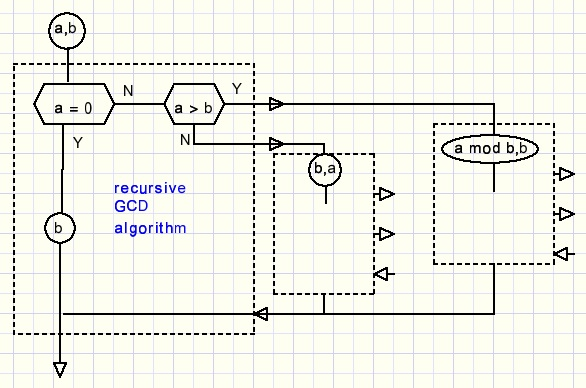a recursive GCD algorithmdownload GCD programdownload Delphi-7 GCD project

It is based on Euclid's theorem which simply amounts to:
GCD(a,b) = GCD(a-b,b)
Example:
GCD(60,45) = GCD(15,45) = GCD(45,15) = GCD(15,15) = GCD(0,15) = 15.

Shorter:
60 mod 45 = 15, GCD(60,45) = GCD(15,45) = GCD(45,15)
45 mod 15 = 0, GCD(45,15) = GCD(0,15) = 15.

Recursive procedures or functions may call themselve.
This allows for compact coding at the price of difficulty to understand.
An approach is to visualize such a procedure many times, see picture below:Here is the function:
```function GCD(a,b : Int64) : Int64;
//b <> 0
begin
if a = 0 then result := b
else if a < b then result := GCD(b,a)
else result := GCD(a mod b,b);
end;
```
That's all !
The program allows for GCD calculations of number up to 12 digits. For details please refer to the source code.